# Probability: Cards vs Dice

#### (A new question of the week)

A couple recent questions involved related subtleties in probability and combinatorics. Both were about apparent conflicts between similar problems involving cards and dice.

## Cards vs dice

This very detailed question came from Alexander in early July:

Hello!

I have a question regarding the probability of getting one pair in poker and Yahtzee. More precisely, I am having trouble with the phenomenon called double-counting, when the same event is counted more than once. I know how to calculate the probability of getting a one-pair in poker. However, when I try to calculate the probability of getting a one-pair in Yahtzee, I get an incorrect answer which I believe has to do with my confusion with double counting. Therefore I will first calculate the probability of getting a one-pair in poker, then do the same thing for Yahtzee and hopefully, you can observe where I went wrong.

Before I show the rest of the question, I want to mention that he is not concerned with how one actually gets the cards in poker or the numbers in Yahtzee, which in both cases can involve more than one step and complicate the probabilities. It will just be assumed that in poker, 5 distinct cards are chosen randomly from a deck of 52, and in Yahtzee, 5 six-sided dice are rolled once.

In poker, one pair means a hand containing two cards with the same number (here, 5), all other cards having different numbers: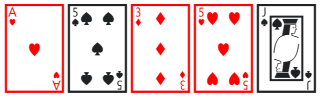To my knowledge, “one pair” plays no role in Yahtzee, but would mean exactly two of one number (5 again), with all other dice having different numbers: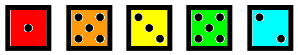As for double-counting (or overcounting), we’ve mentioned it, for example, in Permutations and Combinations: Undercounts and Overcounts, Arranging Letters with Duplicates, and Interpreting and Solving a Counting Problem. It means that we have counted the same outcome more than once, and need to either compensate, or find a way to avoid it. The key is to recognize whether you’ve done it!

Now, Alexander carefully showed his thinking about each problem:

Poker (An ordinary deck of cards with 5 cards in a hand):

(C(13,1) x C(4,2) x C(12,1) x C(11,1) x C(10,1) x 4^3)/3!

First, I take 1 of the 13 values in which I have a pair, and their suit can be combined in C(4,2) ways. Then I choose the third, fourth and fifth cards, all of which can be combined with 4 suits, thus 4^3. To account for double-counting, I divide by 3!. For example, if my third card is six of spades, my fourth is seven of spades and my fifth is eight of spades, that is the same hand as if my third card was eight of spades, my fourth card was seven of spades and my fifth card was six of spades. These three cards can be arranged in 3! ways, and since order does not matter, I divide by 3! to remove duplicates. Finally I divide with the total number of hands which is C(52,5) and get approximately 42%. Now on to Yahtzee.

Yahtzee (5 6-sided dice):

I want to use the same method I did when I calculated the poker-version.

(C(6,1) x C(5,2) x C(5,1) x C(4,1) x C(3,1))/3!

I choose one value out of six, choose two dice then pick the last three dice. Then, the same way I did in poker, I divide with 3!, since a composition of 5-5-1-2-3 is the same as 5-5-3-2-1. Then I divide with the total number of dice-rolls which is 6^5. However, this answer is wrong. To correct my mistake, one would have to remove the 3!, but how then do you account for double-counting? If you could help me understand why I should ignore double counting in Yahtzee, but not in Poker, I would be grateful.

Best regards,

Alexander

He demonstrated the overcounting with cards; is there any with dice?

Hi, Alexander.

Nice question!

I’ll start out by saying that my first impression was that cards and dice behave very differently (because there’s only one of each card, while dice are independent), so I didn’t think the work would be anything like the same. And I think the ultimate answer is going to be that you are being fooled by a superficial similarity between them. Since subtlety is a primary characteristic of combinatorics, that makes this a really nice question to dig into!

Cards are discrete objects, so that there is only one of each; this makes permutations and combinations (which involve selecting and/or arranging distinct items) appropriate. Once you have a four of diamonds, you know the next card can’t be the four of diamonds (though it could be another four). On the other hand, they can be thought of either as ordered (the order in which you get them) or not (just the set in your hand), so either permutations or combinations can be used.

Dice don’t mind having the same number in different places; so there is no permutation involved. Their values are independent. On the other hand, each die is distinct; we often emphasize this by imagining each die being a different color, or tossing each die in a separate place. So in some sense there is an inherent order to them.

But in combinatorics, each tool might be used in any problem, as we’ll see. The two problems do turn out to involve similar-looking work after all.

### Five cards, one pairSo first, let’s think about exactly what you are doing with the cards:

(C(13,1) × C(4,2) × C(12,1) × C(11,1) × C(10,1) × 4^3)/3!

Restating your explanation, you are doing this:

• Choose which number to have a pair of: C(13,1) ways

• Choose which two suits the pair will be: C(4,2) ways

• Choose distinct numbers for the three other cards, in order: 12×11×10 ways

• Choose the suits of these three cards: 4^3 ways

• Account for the fact that order doesn’t count, by dividing by 3!

This counts subsets of cards, not taking order into account; so you divide by the number of ways to choose an unordered subset of 5 cards, C(52,5).

Most of the combinations in his work don’t really need to be written that way; we could simply say that there are 13 numbers to choose from, then $${4\choose2}=6$$ pairs of suits, and then $$12\times11\times10=1320$$ ways to choose three numbers from the 12 remaining, and 4 choices for each of those suits. Then he divides by the number of ways to arrange the same three cards, since everything else is combinations.

He finds the probability as the number of sets of five cards that contain a single pair, over the total number of sets of five cards; probability can be calculated as combinations over combinations, which works best here, or as permutations over permutations.

I had one optional suggestion, letting combinations eliminate the overcount:

The one difference in my own way of thinking is that (12×11×10)/3! can also be thought of as choosing a subset of 3 of the remaining 12 card numbers, namely C(12,3). So the calculation can be alternatively written as

C(13,1) × C(4,2) × C(12,3) × 4^3 = 1,098,240

Dividing this by C(52,5) = 2,598,960, we get 0.4226.

This is all correct; I even checked the answer before moving on, by looking it up here:

https://en.wikipedia.org/wiki/Poker_probability#Frequency_of_5-card_poker_hands

Here is what that page says: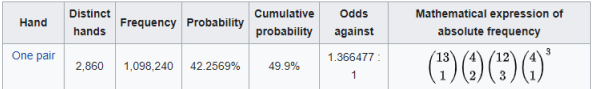Their calculation is identical to ours, not just the number.

Now, why does order not count?

Mostly because none of the choices we made involved locations of the cards; we just chose which cards to include. It would, in fact, be possible to do the work taking order into account. We might do it this way:

• Choose which number to have a pair of: 13 ways
• Choose which two cards will have that number: C(5,2) ways
• Choose a suit for each of them, in order: P(4,2) ways
• Assign numbers to the three other cards, in order: 12×11×10 ways
• Assign suits to these three cards, in order: 4^3 ways
• Divide by the number of ways to select 5 cards in order, P(52,5)

This gives $$\frac{13\cdot{{5}\choose{2}}\cdot_{4}\!\text{P}_{2}\cdot12\cdot11\cdot10\cdot4^3}{_{52}\text{P}_{5}}$$ $$=\frac{13\cdot10\cdot4\cdot3\cdot12\cdot11\cdot10\cdot64}{52\cdot51\cdot50\cdot49\cdot48}$$ $$=\frac{131,788,800}{311,875,200}\approx0.422569,$$ just as before. The important thing is consistency: If any calculation takes order into account, then all must.

### Five dice, one pairNow, how about the Yahtzee version? (I took a moment to check the rules for Yahtzee, which I probably haven’t played in 50 years, and decided that you are just talking about rolling 5 dice once, and seeing if there is a single pair, without doing all you might do in a real game.)

The same basic strategy does apply, but the details are different. We are no longer counting subsets; in your denominator, 6^5, order is taken into account! That is what you missed.

(Note that it would be very hard to solve a dice problem without taking order into account, because duplicates are allowed, so rearranging would not always change the result.)

This is an important point; students often ask how you know whether order does or does not matter, and often in probability questions, that is up to you to decide. As long as the possibilities you count are equally likely, you can calculate probabilities as a ratio of permutations or of combinations. In a hand of cards, as I showed above, you can either think in terms of how the cards are dealt (permutations: order matters) or the set of cards you have in your hand (combinations: order doesn’t matter – or you might always sort them by number and suit, ignoring the order in which you got them). With dice, you generally want to think as if each die were distinguishable (different colors, say), which means that order (which die has which value) matters. You could conceivably ignore order, but it would be very hard to count, and what you counted would not be equally probable. (As a simple example, when you toss two coins, the only possibilities ignoring order are HH, HT, TT, but HT happens half the time.)

So we choose, with good reason, to think of a roll of the dice not as a set of numbers (e.g. {1, 1, 2, 4, 4} – but that would actually be a multiset), but an ordered list of numbers (e.g. 2, 1, 4, 4, 1). On the other hand, this does not make it a permutation, because duplicates are allowed. There are $$6^5=7776$$ of these, not $$_6\text{P}_5=720$$.

So here is what you are actually doing:

• Choose which number to have a pair of: C(6,1) ways

• Choose which two dice the pair will be: C(5,2) ways

• Choose distinct numbers for the three other dice, in order: 5×4×3 ways (that is, P(5,3)

We don’t need to divide by 3!, because this time order does count. Our result is

C(6,1) × C(5,2) × P(5,3) = 3600

Dividing this by 6^5 = 7776, we get 0.4630 (46.3%).

Note that where we chose two suits last time, we are choosing two dice to have the chosen numbers: superficially similar calculations, but entirely different in meaning.

Alexander had calculated $$\frac{{6\choose1}\cdot{5\choose2}\cdot{5\choose1}\cdot{4\choose1}\cdot{3\choose1}}{3!}=\frac{6\cdot10\cdot5\cdot4\cdot3}{6}=600,$$ which amounts to $${6\choose1}\cdot{5\choose2}\cdot{5\choose3},$$ whereas we really have $${{6}\choose{1}}\cdot{{5}\choose{2}}\cdot _{5}\!\text{P}_{3}=6\cdot10\cdot60=3600.$$

When I work a problem like this, I like to first ask, What am I counting, and then, How can I count them? In this case, because we have just done a very different problem that was misleadingly similar, the first question was the key.

Alexander replied,

Thank you!

I have searched everywhere to find an explanation like yours, but I have not found one until now. Again, thank you!

## Poker hands with random numbers

This week, while I was editing this post, we got a question that deals with a very similar problem. I normally let a question sit for a month before publishing it (to make sure the discussion is finished, and in some cases to make sure an assignment will be past due before showing the answer to the world), but this belongs here.

### Full house of cards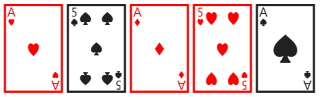Bijan wrote, first quoting from a book (Probability and Statistics with Applications: A Problem Solving Text, by Leonard Asimow and Mark Maxwell) how to calculate the probability of a full house (3 of one denomination, and 2 of another) in poker: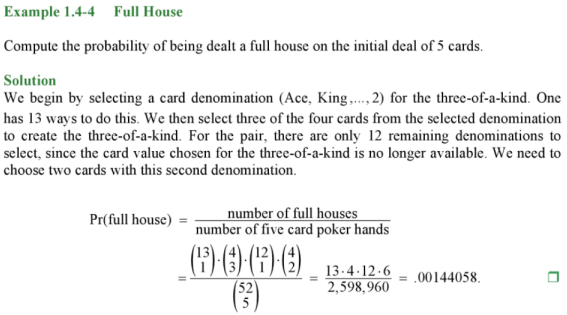Then he explained that he was trying to find the probabilities for the “poker test” for random numbers, which treats each sequence of 5 digits produced by a random number generator as a “poker hand” and compares the experimental probabilities of various “hands” to those expected for truly random numbers. He included a link that says this: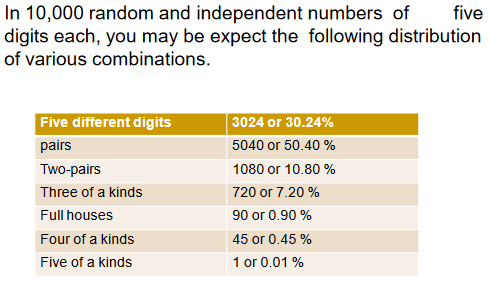### Full house of digits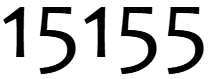Then he showed his work for each case, starting with the full house:

We’ve 10,000 random numbers of five digit each. They’re assumed to be independent.

My calculations-:

1) Full house

10C1*9C1/10,000 = 0.009

I’m correct. My only confusion here would be the denominator. Why is it 10,000?

According to the above example, should not it be 10C5?

Explanation of my thought process-:

First pick 1 digit out of 10 digits. Then next, pick another digit(only 1 digit as we need a pair), out of remaining 9 digits.

I’ll quote more cases later. I answered this part:

Hi, Bijan.

It happens that the blog post I’m working on for this week is about almost exactly the same issue: the difference between problems about cards and about dice, which amount to random number generators. A central difference is that cards are unique, but digits can be repeated; so you tend to use permutations and combinations for cards, but not so much for numbers (and for different reasons). Another central difference is that order is ignored in poker hands, but not in numbers.

Even more clearly than dice, random numbers have a definite order of digits; 11234 is not the same as 21314.

Let’s look at your first case, the full house, to see whether you got the right answer rightly or by accident.

First, the denominator should not be 10C5, as you suggest, because you are not choosing 5 different digits ignoring order, but 5 unrestricted digits counting order!

It should be 10^5, which is 100,000. I have no idea why you said 10,000.

Later I realized what he had done:

Ah! I just looked at the link you put at the end; I see it says

In 10,000 random and independent numbers of five digits each, you may expect the following distribution of various combinations

That is not the number of possible numbers; they just chose to suppose that you have that many numbers in your sample, for the sake of the table following. You forgot to stop and think about where that number would have come from, and whether it made sense as you interpreted it.

In the table, each probability is multiplied by 10,000, the number of random numbers they suppose we are testing. The correct denominator is the number of possible 5-digit numbers, which is $$10^5=100,000$$, counting all numbers from 00000 to 99999.

But that’s not the only error. Continuing,

As for the numerator, I would simply say we choose one of 10 digits to occur twice, and one of the remaining 9 digits to occur three times. But then we have to place them in some order, because order does count. So we choose 2 of the 5 places to put the first digit we chose, which we can do in 5C2 = (5*4)/(2*1) = 10 ways. So the probability of a full house is (10*9*10)/100,000 = 0.009.

The error of neglecting order was corrected by the error of using 10,000 as the denominator!

I would write it as $$P(\text{full house})=\frac{_{10}\text{P}_2\cdot{5\choose2}}{10^5}=\frac{10\cdot9\cdot10}{100,000}=0.009=0.9\%.$$

### Four of a kind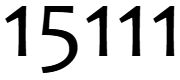He made the same error for one pair and for three of a kind, thinking he was right because of an error hidden by the wrong denominator. That compensation failed in his work for four of a kind:

4) Four of a kind:

So from 10 digits, I need to pick 1 digit and out of the remaining 9 digits, I need to pick another 1 digit.

So, it should be 10C1*9C1/10,000 = 0.009

But it becomes similar to full house. This is wrong. I don’t get why this became wrong.

I didn’t directly answer this, but he has again omitted the choice of a location for the four, which can be done in $${5\choose4}=5$$ ways. The correct answer is $$P(\text{four of a kind})=\frac{{10\choose1}\cdot{9\choose1}\cdot{5\choose4}}{10^5}=\frac{10\cdot9\cdot5}{100,000}=0.0045=0.45\%.$$

### All different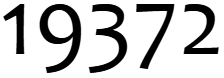For the last two cases he did, “all different” and five of a kind, the only error turned out to be the denominator. Here is the former:

5) 5 different digits:

This should’ve been simple, I got the answer but I got the answer greater than 1.

10C1*9C1*8C1*7C1*6C1/10,000

=3.024

I’m not sure why I got this. I am skeptical about the denominator since the start as I feel that’s randomly chosen here unlike above where we did 52C5. If I increase 1 “zero” in denominator, the answer would be correct.

This is a case where I would use permutations, but not in the same way as for cards. The numerator will be the number of ways to form a number consisting of 5 different digits, which means a permutation of 5 of the ten possible digits. That is

10P5 = 10*9*8*7*6 = 10!/5! = 30,240.

The denominator, again, is the number of ways to choose 5 (non-distinct) digits, which is just 10^5 = 100,000, since there are 10 independent ways to choose each. So the probability is

30,240/100,000 = 0.3024.

That is, $$P(\text{all different})=\frac{_{10}\text{P}_5}{10^5}=\frac{10\cdot9\cdot8\cdot7\cdot6}{100,000}=0.3024=30.24\%.$$